# Small PA driver for HF

Under construction...

Small PA driver for the Hermes-Lite Class-A HF PA.
Circuit is based on a classical resistive feedback topology.

Main characteristics:

• 14 dB gain
• 180 mW (22.7 dBm) output at 1 dB of compression
• 50 mW per output tone for an IM3 of -30 dBc with a two-tone input
• DC input power: Vdc=12.0 V, device bias current IccQ=42 mA

Schematic: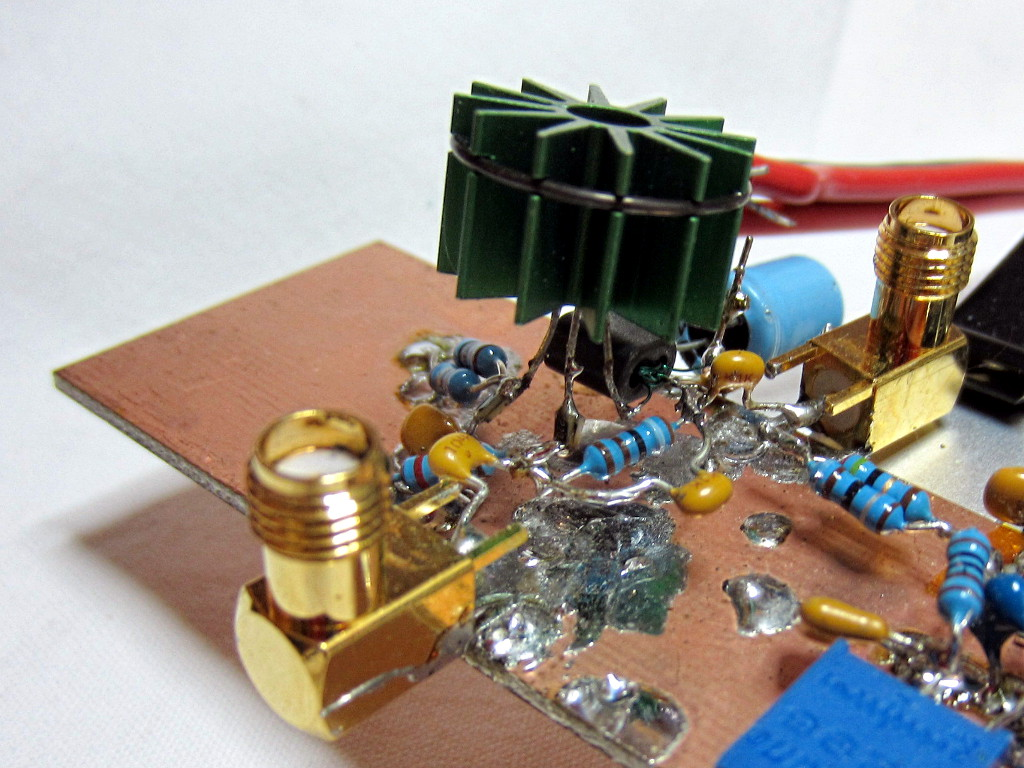Intermodulation products at 190 mW PEP output:Measured small-signal S-parameters (with -16 dBm input):.

The fundamental and harmonics output levels versus input power, with a 10 MHz sinewave input, is shown in the graph below:the output power at 1 dB of compression is about 180 mW (22.7 dBm).

RF output and DC current drawn from the supply versus input power (10 MHz sinewave) are shown in the following graph: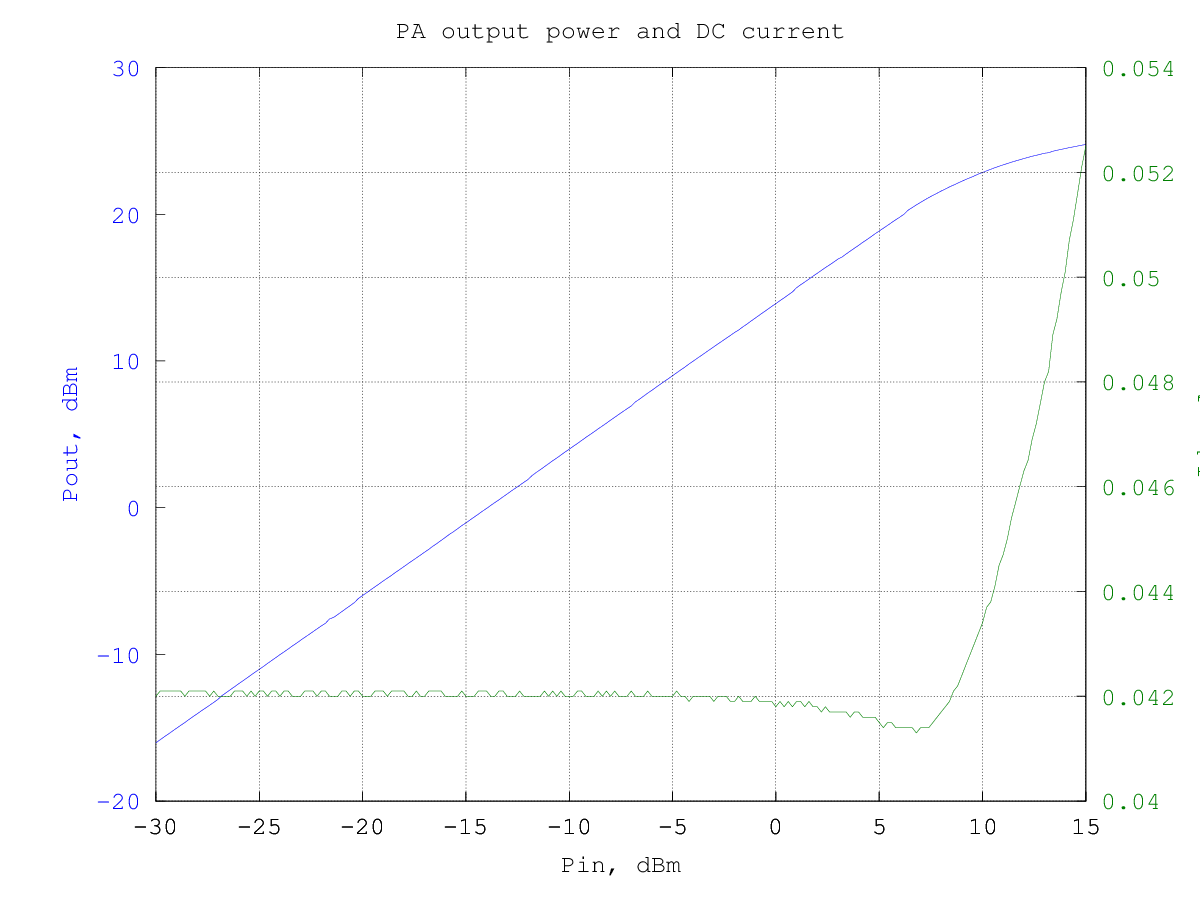and the resulting efficiency is shown in the graphs below (data are the same for the two graphs, in the first the input and output power are in dBm and in the second they are in watt):

The third-order intermodulation products amplitude w.r.t the output power per-tone is shown in the graph below (to obtain the actual [average] output power multiply the value by two, to have the PEP, multiply by 4):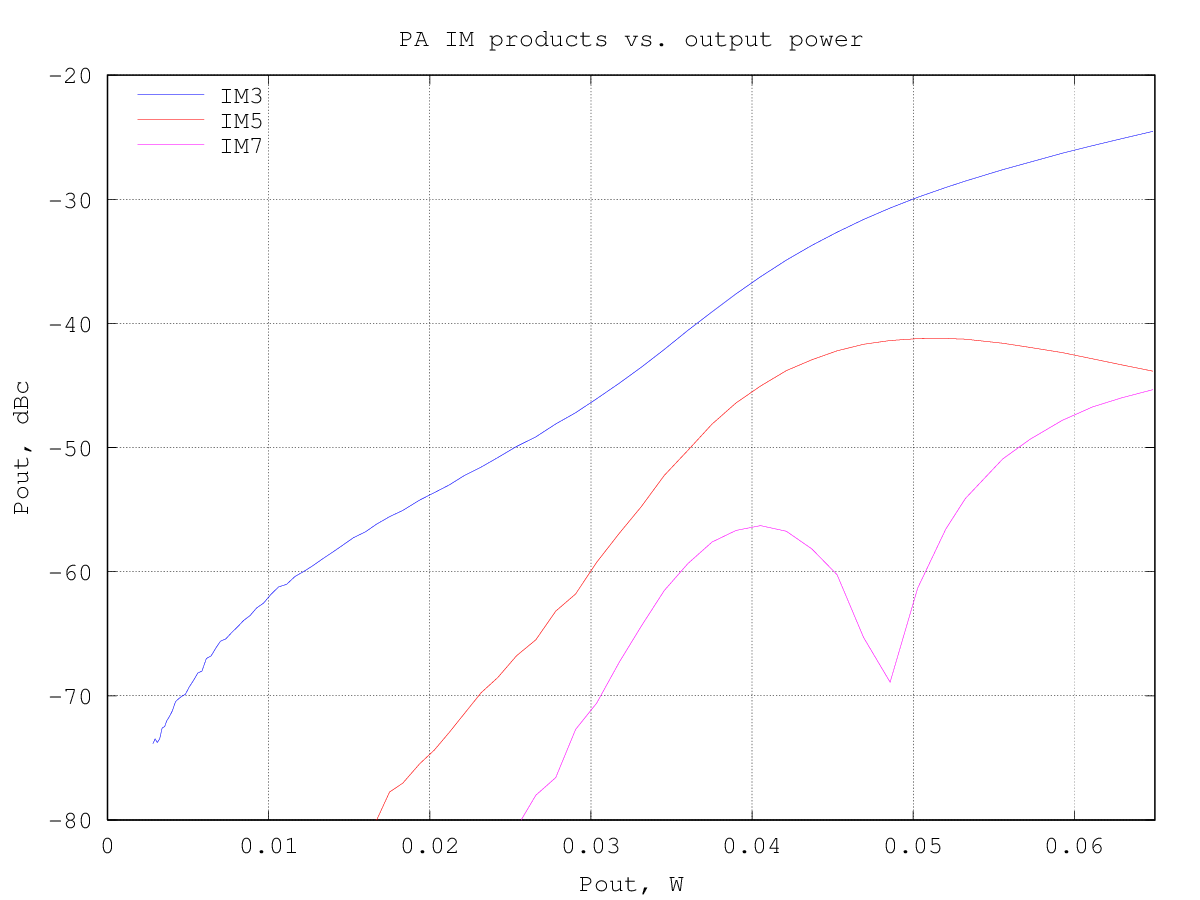at an IM3 of -30 dBc the output power is around 50 mW per tone.

The graph below shows the relative amplitude of the IMD products (separately for upper and lower products) for a two-tone test with varying tone spacing at 195 mW PEP output: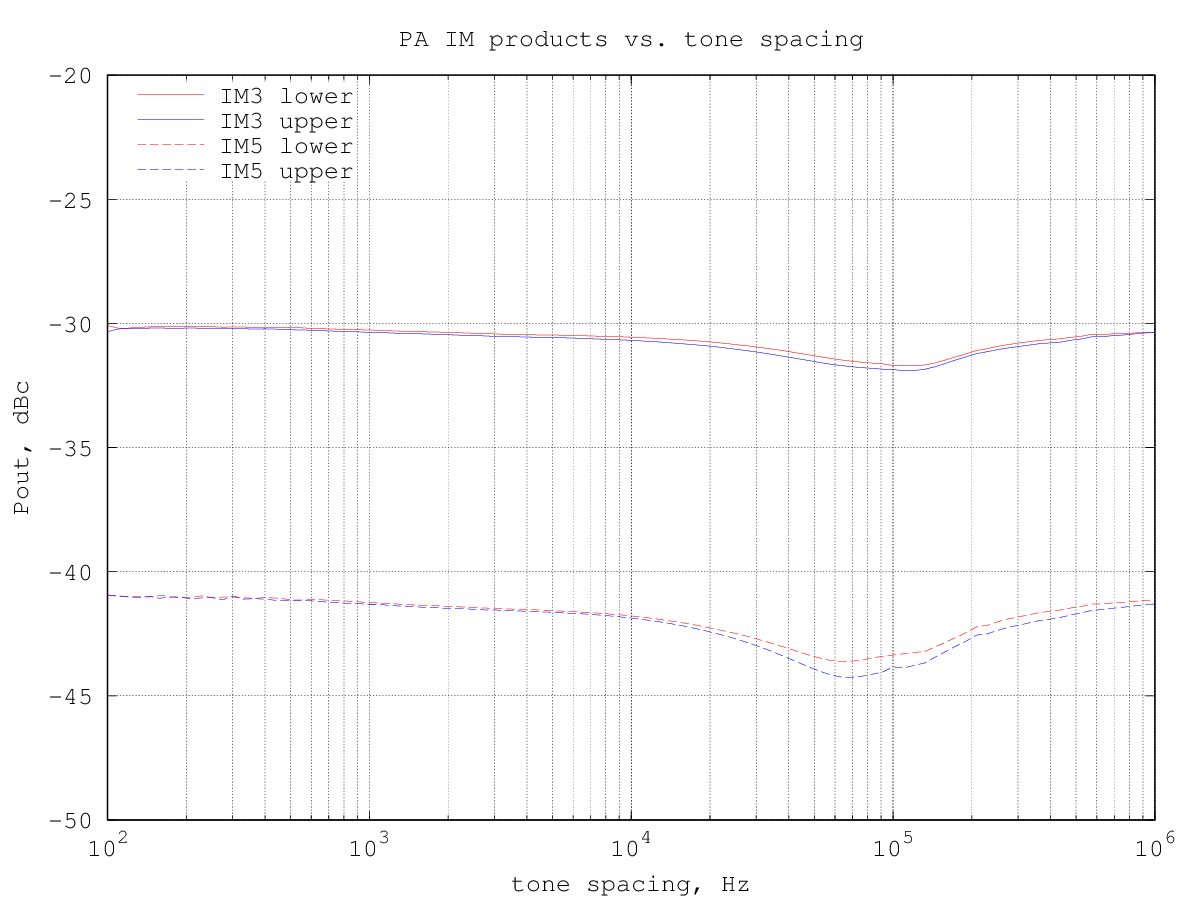By plotting the third-order intermodulation products power vs. the input power we can find the intercept point by extrapolating the results. The amplifier third-order intermodulation products follow the theoretical 3:1 slope w.r.t. the input power up to about 1 dBm input, as can be seen from the graph below: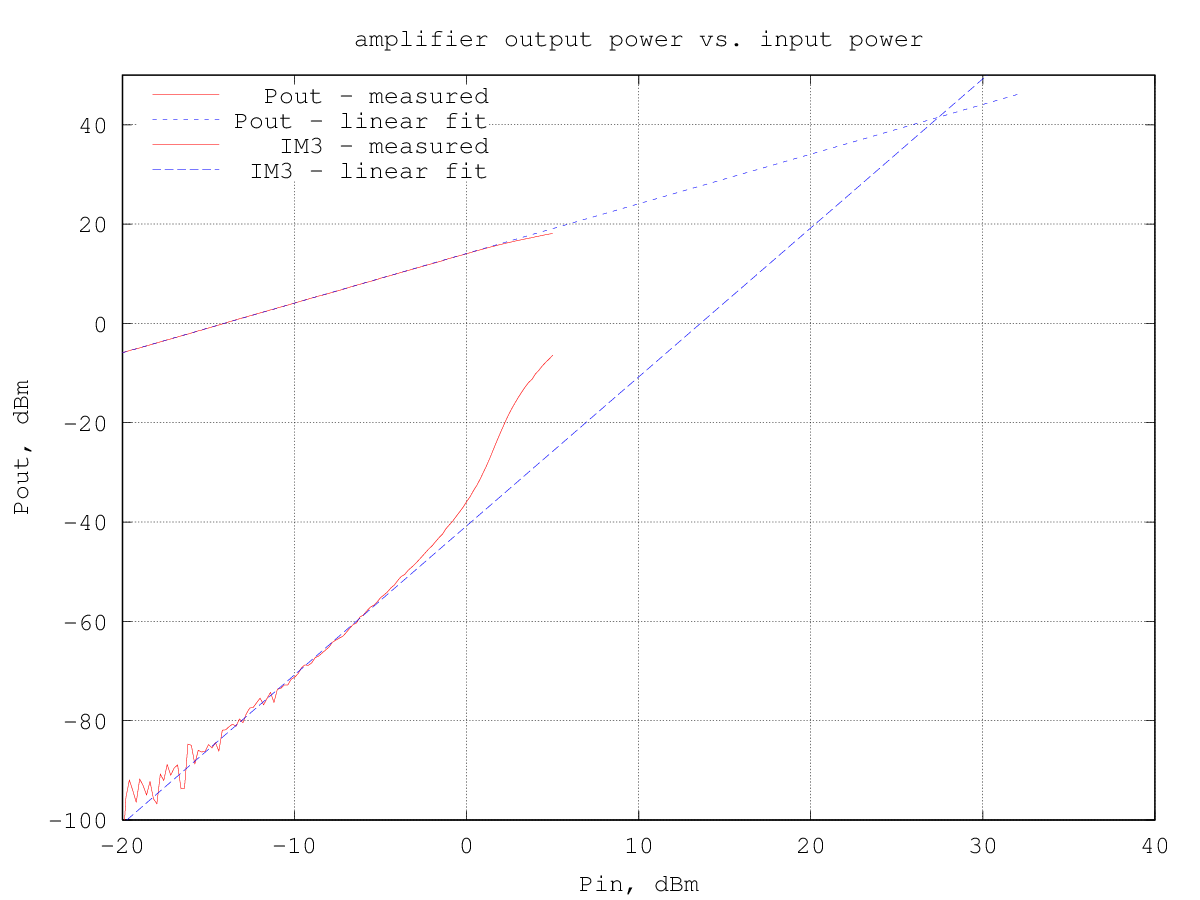The input IP3 turns out to be 27.4 dBm and the corresponding output IP3 is 41.5 dBm.